# Predicting total outcome stats in a MLR season using R (2)

05/11/2020

Almost a year ago, I wrote an article about predicting total outcome stats in a MLR season. I ended up calculating the expected number of expected hits, home runs and strikeouts in a season. It ended up being pretty close to the actual number of the respective outcomes in season 3.

Now, we will repeat that process for the season 4 data and also try predicting the total number of outcomes for season 5. For those of you that don't know or forgot, batting types were updated in season 5. Nevertheless, the math stays the same and you will see that we'll be able to re-use most of the calculations for season 3 and 4.

## Just the Basics

In my last article, I spared you the math and used simulation to calculate the outcomes. This time, we will use exact probabilities. For this, we first have to brush up on some probability theory.

## Statistical Variables and the Discrete Uniform Distribution

A discrete statistical variable X is uniformly distributed if a finite number of values are equally likely to be observed. To visualize this, let's have a look at the probability function of such a variable that takes in values from 1 to 1000 (inclusive):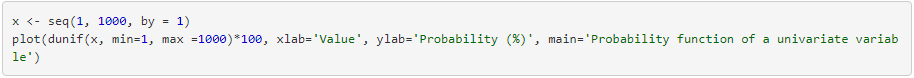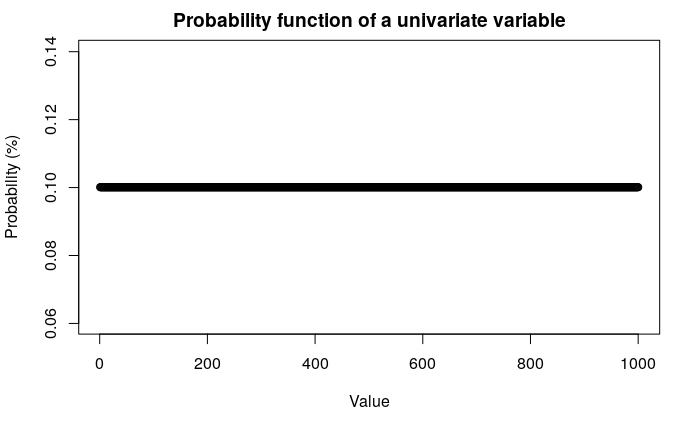Every value from 1 to 1000 has a probability of 0.1% to be observed. Sound familiar? This is what we would expect the pitch and swing variables in fake baseball to be like. Therefore, let's assume the statistical variables Pitch and Swing are ∼U{1,1000} (discrete uniform statistical variables from 1 to 1000).

## Independence of Statistical Variables

We assume that Pitch and Swing are independent statistical variables. Hence, the probability p(pitch,swing), i.e. that we observe a pitch of value pitch and a swing of value swing is the same as p(pitch)⋅ p(swing). Things would get complicated otherwise. Also, for this article, it's sufficient to assume independence.

## The Multivariate Distribution called Difference

Let's recall our definition of the difference between a pitch and a swing from last time: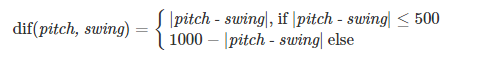We can define this function in R: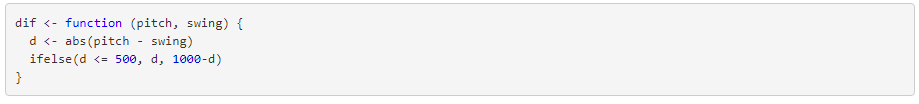We can use this to define a third statistical variable, Diff, which is defined as Diff=dif(pitch, swing). Now, we would like to calculate the probability of each possible difference. Differences are values from 0 to 500 - there are 501 different values for differences. We can calculate the probability of each diff happening by calculating the differences of all possible pitch and swing values and counting how often each difference occurs and multiplying that with the probability that pitch and swing occur together, which is 1/1000⋅ 1/1000. Let's look at the R code: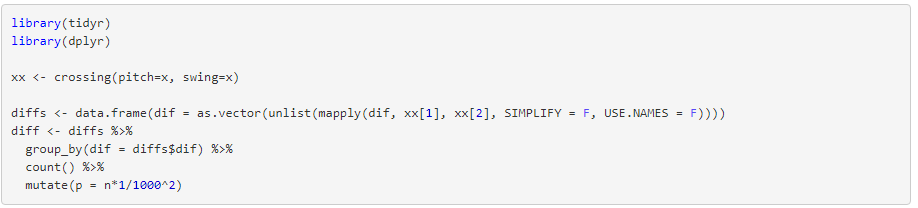We first generate all possible pairs of swing and pitch by using the cartesian product of a vector (1,2,...,1000)ᵀ. This results in a 2 by 1,000,000 matrix. We then apply the dif function to each pair and assign it to the diffs variable (a vector of length 1,000,000). Then, we count the occurence of each difference and multiply it by 1/1000⋅ 1/1000  - this is the probability of the difference occurring. We store the diff, the number of occurrences and the probability in a matrix (a data frame). It looks like this: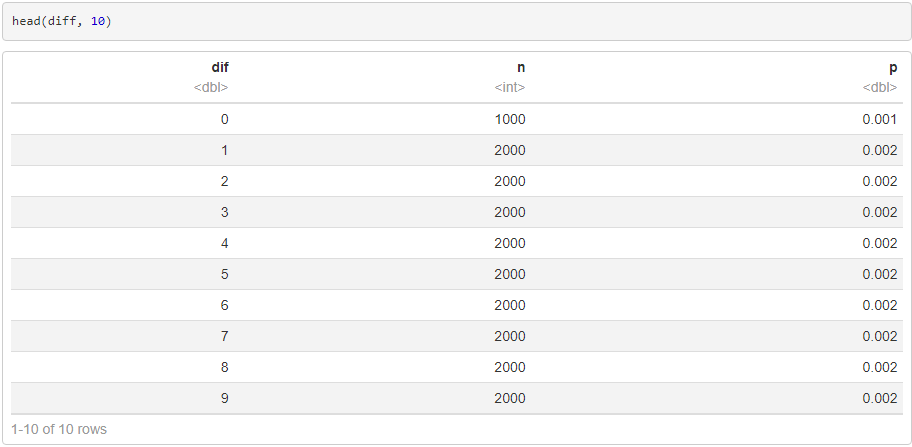Let's visualize the probability function: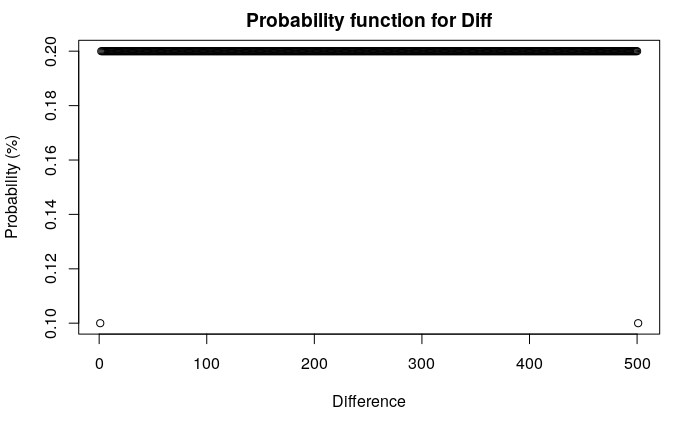Each difference has a probability of 0.2% to occur, except for 0-diffs and 500-diffs, which only have a probability of 0.1% to occur. You may ask yourself now why we have different probabilities for 0 diffs and 500 diffs. It may be easier to understand if we look at the possible differences for a pitch of value 1: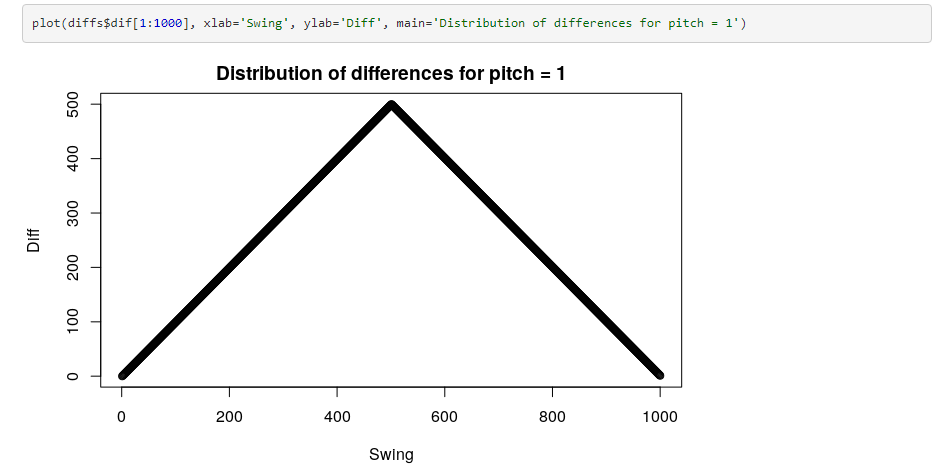We can see that for each swing that we can make for a pitch of 1, every difference can occur twice except for 0 and 500. Therefore, the probability that we observe a difference of 0 or 500 is lower. This of course extends to all other combinations of pitches and swings. Knowing all that, we could simplify our calculations in the following way:

Given a pitch and swing, the probability of each difference that is not 0 or 500 is 2/1000⋅ 1/500. For the differences 0 and 500, the probabilities are  1/1000. Since this is true for any pitch and swing, these are the probabilities for all combinations of pitches and swings. In short, there is no need to count like we did, since the wraparound of the difference calculation simplifies things quite a bit. Both approaches are valid.

We can also calculate the expected value for Diff, which is given by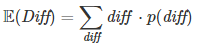In R: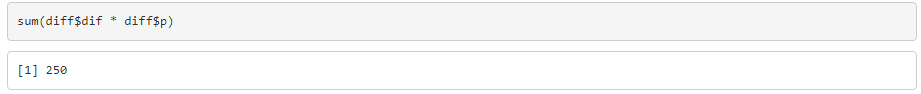As expected, the expected difference between a pitch and swing is 250. If you remember, last time we got a expected difference of 249.9 with simulation.

## A Look Back: Season 3 Outcomes

Now that we have the probability function for Diff, we can re-do the calculations for the number of hits, home runs, and strikeouts in a season. Again, we assume that we play in a neutral park and we do not consider the number of players with a specific pitcher/batting type. We average the ranges for all possible pitcher-batter combinations in season 3 to get estimates for the average range.

In this section, we will not re-do all the calculations from my previous article. We'll just have a look at the probabilities for a home run, a hit and a strikeout.

## Home Runs

In my last article, we estimated a probability of about 4.7% that a home run occurs. We assumed an average home run range of 0-23. In order to calculate the exact probability now, we can use the addition theorem on probability to calculate the probability of the event: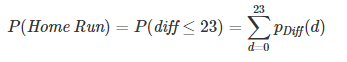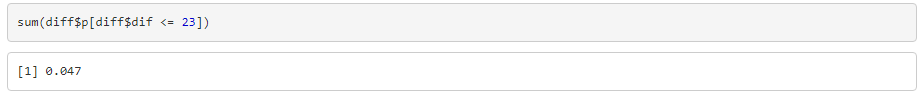And look at that, the probability is exactly 4.7% that the difference between a pitch and swing is between 0 and 23.

## Hits

We repeat the process from before. This time, we calculate the probability of a difference between 0 and 109.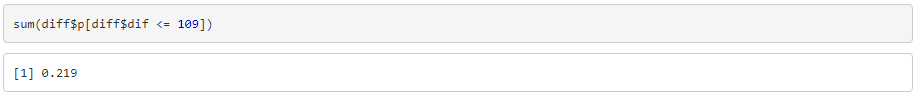It turns out to be 21.9%, which is also what we got last time. On to Strikeouts.

## Strikeouts

The only difference here is that for strikeouts, the range does not start with 0, but with 247. The upper value for the average strikeout range was 341.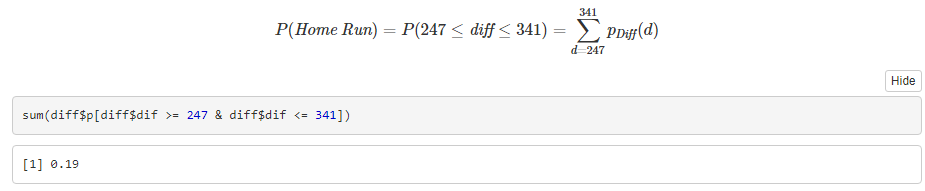This is quite exactly the same result we got in the previous article.

## A Not So Far Look Back: Season 4

What changed between seasons 3 and 4? Well, the league expanded to 30 teams. Batting types stayed the same. Hence, we just have to adjust total outcome estimates by the number of at-bats in season 4. There were 11722 plate appearances recorded in the regular season of season 4 according to the MLR S4 roster sheet.

## Home Runs

The probability of a home run with our batting types from season 3 is 4.7%. So let's just estimate how many home runs there should have been in season 4: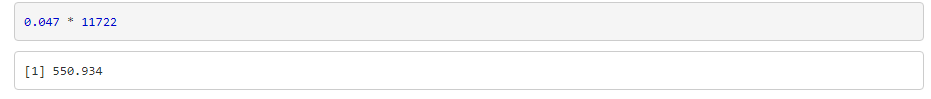We get about 551 home runs, which is 12 more than were recorded. Not as good of an estimate as last season, where we were only off by 3.

## Hits

Same thing again, but this time with a probability of 21.9%: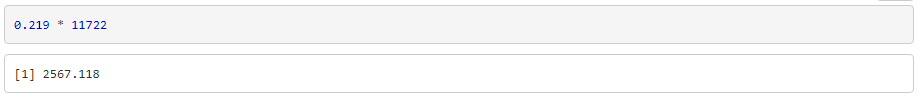Wow, we're off by a mile here. There were 2703 hits recorded in season 4. We underestimated the number of hits by 136. I'm starting to feel uncomfortable.

## Strikeouts

Alright, new outcome, new chance. Let's use our 19% probability for a strikeout and see what happens: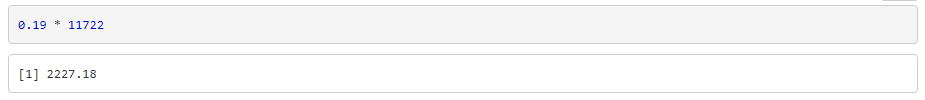Alright, the number of strikeouts in season 4 was 2254, so we are only off by 27 strikeouts.

## Intermediate conclusion

These results leave a sour taste in my mouth. Why did our predictions worsen compared to last season? Maybe our approach was not correct. I went back to the season 4 roster sheet and saw that master statistician Daniel Dove actually made an average result range based off player types in the roster sheet. His average ranges are based off the basic balanced pitching type and the basic neutral pitching type and average hand penalties. Let's see if those estimates are better than ours.

## Home Runs - part 2

Daniel Dove's average Home Run range is 0-22. Let's see how that affects our estimate: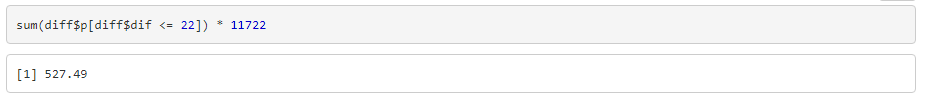With Dove's average range, we underestimated the number of home runs by 12. This makes sense, as we reduced our original range by 1.

## Hits - Part 2

The average hit range according to Daniel Dove is 128, much larger than our estimate of 109. Let's see if the estimate with the larger range is better: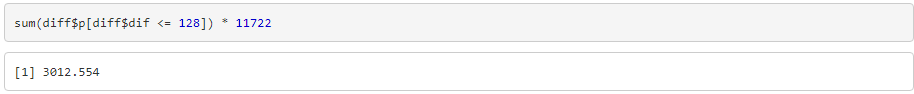Not really. We now overestimate by 305 hits. This is not better than before.

## Strikeouts - Part 2

Daniel Dove's average strikeout range is between 277 and 373. Let's see if the strikeout estimate is better: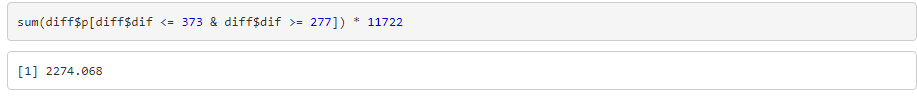This estimate is marginally better, overestimating the number of strikeouts only by 20.

Apart from the gross overestimation of hits, the League Statistician's average ranges were better, but only slightly. We'll stick with our estimates for the rest of the article.

## Putting it into perspective

In absolute numbers, our estimates for the number of home runs, strikeouts and hits were worse. But how do they compare in relative numbers? Remember, there were only 9455 plate appearances in season 3. Let's have a look at the percentages: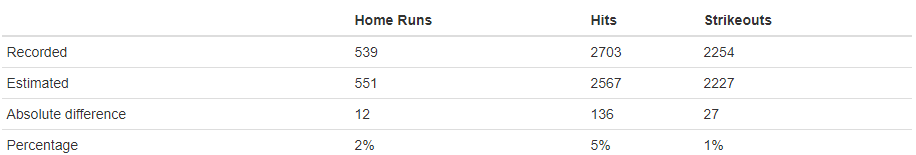This is indeed a bit worse than last season, where our error was within 1% for all outcomes. However, it's not as bad as the actual numbers would make it seem.

## A Look Ahead - Season 5

Alright, this is where we'll make a projection. Last season, I did not do this because I expected that predicting the number of plate appearances for season 4 would be a pain. We did not expand MLR in season 5, hence we'll expect that the number of plate appearances will stay the same. However, batting types have changed in season 5, so we'll have to calculate new average ranges. I did the same thing as last time, the Google doc with the range calculations can be found here. This season, we have 729 different pitcher-batter combinations. As before, we assume that pitcher-batter combinations are evenly distributed in the league and we play all games in neutral parks.

The average Home Run range we get by averaging all possible ranges is 23.59. We'll use 24. For hits, our new average range is 112, compared to season 3's 109. The strikeout range changes the most, as it is now between 249 and 334. Let's estimate the number of occurrences for each outcome and summarize them in a table.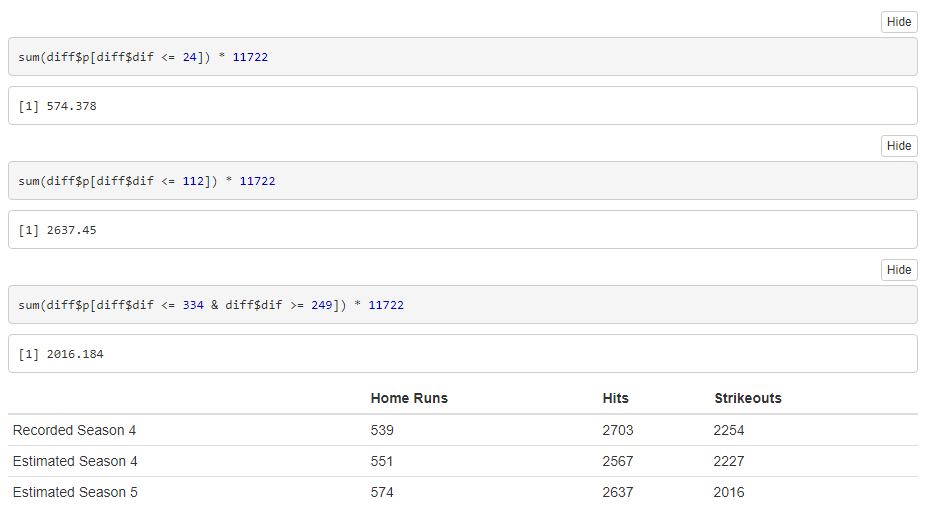With the new batting and pitching types/ranges, we expect the total number of home runs and hits to increase and strikeouts to decrease in season 5. Since the number of hits in season 4 was quite a lot higher than we expected, it will be interesting to see if the new types will actually lead to the expected changes in total outcome numbers. The biggest factor here is probably the number of plate appearances, but I can't be bothered to come up with an estimate for that. Maybe one of you can come up with a better prediction for the number of outcomes.